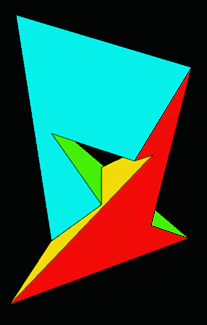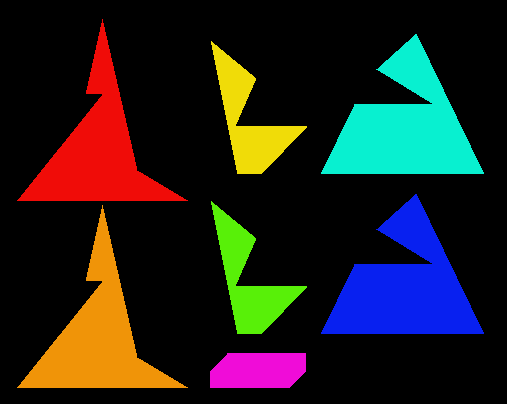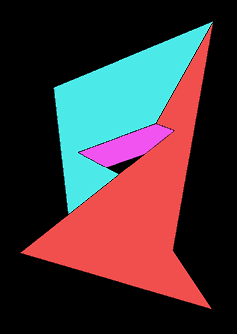the Szilassi polyhedron

In 1977, Hungarian mathematician Lajos Szilassi found a way to construct a toroidal heptahedron. Each face of his polyhedron is a hexagon (although none of them is a regular hexagon).

To make your very own model of a Szilassi polyhedron, click here to download a pattern in PDF (52807 bytes, viewable with the free Adobe® Acrobat® reader ). For aesthetic reasons, I've altered the polyhedron's angles and proportions slightly from Szilassi's originals. Click here for Dr. George Hart's tips on constructing paper polyhedron models. (And if you make a polyhedron with this pattern, feel free to let me know how it came out. I'm happy to hear from people who've checked out this web page.)

The Szilassi polyhedron shares with the tetrahedron the property that each of its faces touches all the other faces. A tetrahedron demonstrates that four colors are necessary for a map on a surface topologically equivalent to a sphere; the Szilassi polyhedron demonstrates that seven colors are necessary for a map on a surface topologically equivalent to a torus. (Neither demonstrates anything about how many colors are sufficient.)

The vital statistics of the two polyhedra are as follows:

 tetrahedron Szilassi polyhedron 4 faces 4 vertices 6 edges 0 holes 7 faces 14 vertices 21 edges 1 hole

What other kinds of polyhedra could have the property that each pair of faces shares an edge? Maybe none.

Euler's formula f + v - e = 2 is easily generalized for polyhedra with holes;
if h is the number of holes, f + v - e = 2 - 2*h .

In a polyhedron every pair of whose faces shares an edge,
f and e are related by the equation e = f * (f - 1) / 2.

Each vertex must be at the intersection of three edges. (If there were more than three, some pairs of faces couldn't share an edge.) Thus, as each edge connects two vertices, v = e * 2 / 3.

Combining the above equations and simplifying gives

h = (f*f - 7*f + 12) / 12

which can be factored as

h = (f - 4) * (f - 3) / 12

. Only certain values of f yield whole numbers of holes. f=4 applies to the tetrahedron; f=7 applies to the Szilassi polyhedron. The next value of f that yields a whole value for h is 12, which would apply to a polyhedron with 12 faces, 66 edges, 44 vertices, and 6 holes. That configuration doesn't sound workable to me; higher values of f and h seem even less likely. Obviously I'm a bit short of a proof, but you get the picture. Click here to see a model of the configuration of the dual of such an unlikely polyhedron.

Szilassi gave a set of equations that describe a particular instance of a 7-sided toroidal polyhedron; Martin Gardner reproduced patterns for the faces (in the context of an entertaining discussion about math and minimalist art) in his Mathematical Games column in Scientific American (Nov. 1978). Szilassi's seven faces, in their original shapes, are also illustrated below.

There is a dual to the Szilassi polyhedron. It was discovered in the late 1940s by Ákos Császár and has 14 triangular faces, 7 vertices, 21 edges, and one hole. Patterns for a model of the Császár polyhedron can be found in Martin Gardner's column in the May 1975 Scientific American. (The math above was adapted from Gardner's description of Donald W. Crowe's analysis of the relationships among f, v, e, and h in the Császár polyhedron.)

There's an applet at http://mathworld.wolfram.com/SzilassiPolyhedron.html that shows how the Szilassi polyhedron looks at various rotations that you can control with your cursor. (Last I checked, the Java code in that applet exhibited a bug in its hidden-surface removal methods; the polyhedron isn't rendered properly at certain rotations. The bug isn't a big deal; I found it more amusing than annoying.)

Images on this page were generated by a program I wrote to play with altering the angles and proportions of the polyhedron. To download C++ source and documentation for the program (gzipped tarball, 44379 bytes; requires X Window graphics), click here. If you'd like further information, send me email.

Tom Ace